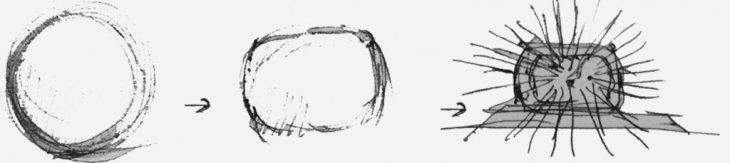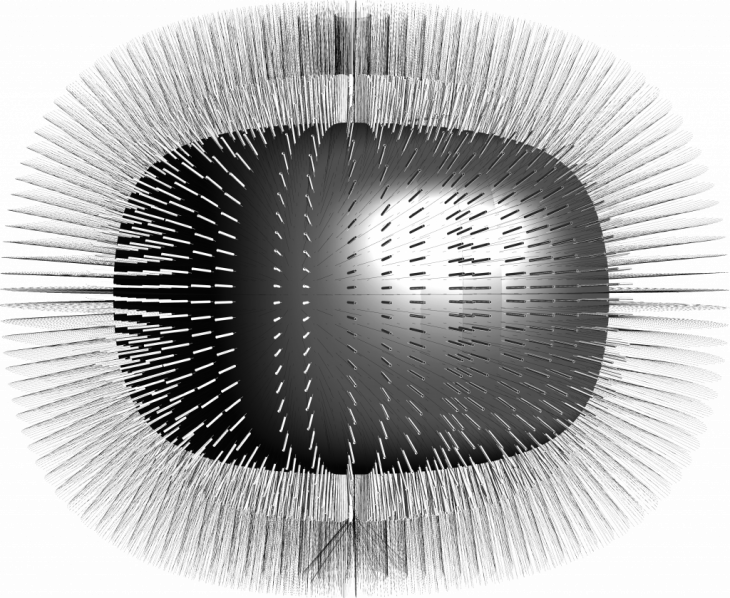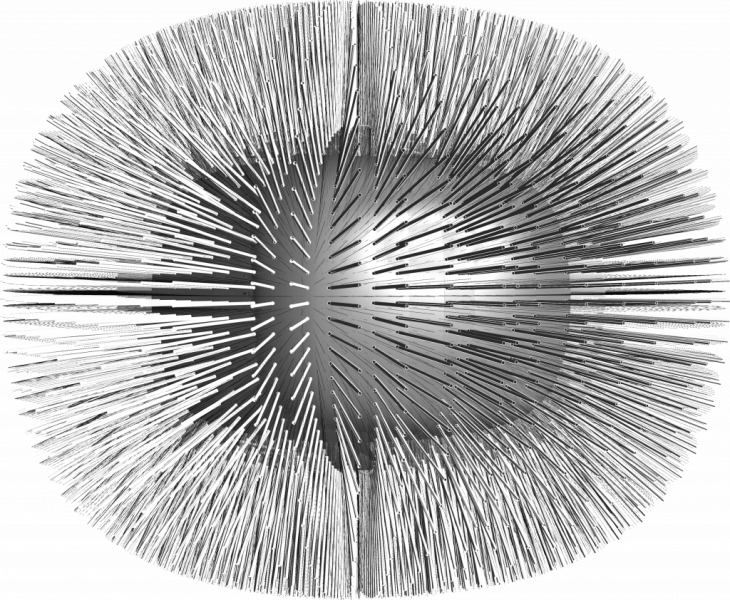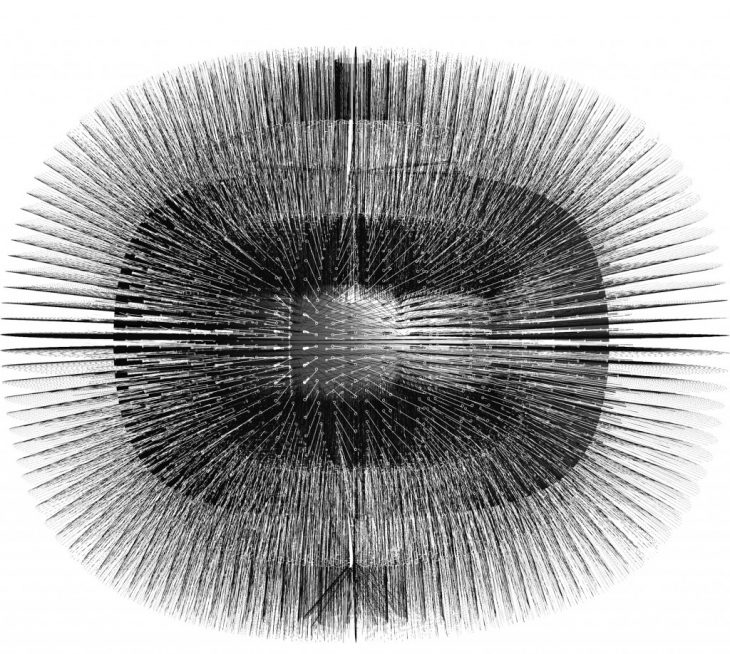The Concept of the Form Development :SPHERE                                         ROUNDED CUBE                            PARAMETRIC RODSr = 20                                             r = 25                                                       r=30

r*sin(sin(sin(v)*cos(u)))

r*sin(sin(sin(v)*sin(u)))

r*sin(sin(cos(v)))/(13/10)

Pseudo code  for  the building envelope :

1 – Makes range for two lists of a number in order to make Sphere the basic geometry.

2- Sorting different (sin, cos) equations as a cartesian system in order to control the points of the
sphere.

3- Deconstruct a point in the 3 axes with different lists in order to match the data of numbers.

4- The 3 basic equations {r*sin(v)*sin(u) , r*sin(v)*cos(u), r*sin(v)} which they form a sphere ,but
in order to make the rounded cube with three modeling methods (flattening-scaling-thickening) should decrease the values of the three equation by making multiplications with the same (sin) value for the whole each equation in each axis.

5- , r*sin(sin(sin(v)*sin(u))) , r*sin(sin(sin(v)*cos(u))), r*sin(sin(cos(v)))/(13/10) these three
equation form the rounded cube.

6- Transfering the deconstructed points to interpolate curves in order to make the skin
of the rounded cube.

7- Dividing the points of the interpolate curves which help to control the rods of the pavilion
or the (SDL) lines which they are controlled through vectors.

8- After transferring the points to the surface we have to evaluate the surface in order to know the
normals of the sphere which helps to control the directions of the rods.

9-The last thing which is forming the rods by sweeping the pipes through the (SDL lines).

10- the (r) value is controlling the density of the rods on the sphere we are going to use
three variables (r=25, r = 30, r = 15).Parametric Values controlling the density of the rods with specifying the form shape

### r                                             U                                                   VThe density of the rods depends on the  (r) value, r = 25  which is a parametric value of the equation. Parametric Skin of Rods  is a project of IaaC, Institute for Advanced Architecture of Catalonia